# Setup for 2 Player a Game of Food Chain Magnate

Originally generated on 10/11/2018 8:10:20 PM
A direct link to this setup is https://www.BoardgameHelpers.com/FoodChainMagnate/LoadGame.aspx?id=\$2a\$04\$bcot4Vk1OLByld5xL08rSe

## Player Setup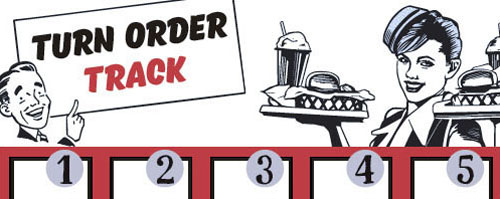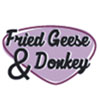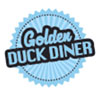## Other Setup

Bank starts with \$100.00
Remove these Billboards: #12, #15, #16
Number of 1x Employee cards used = 1

## Map (Balanced)

Click here to view Map Tile Lettering Key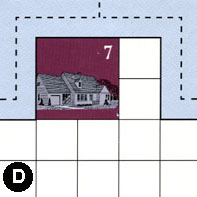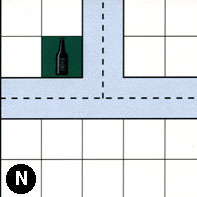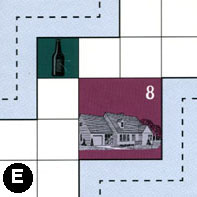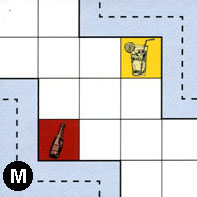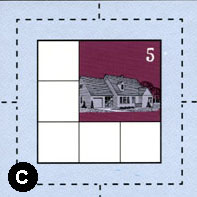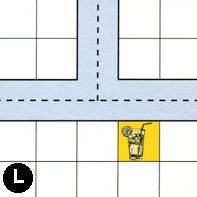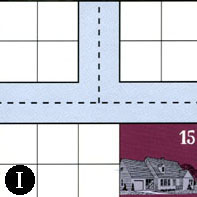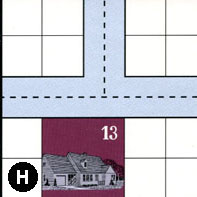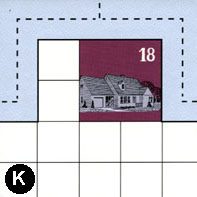### Map Stats

Map Option = Balanced
Balance Issues = Not enough large neighborhoods. Not enough empty space in neighborhoods.
Total Number of Tiles = 9
Total Number of Starting Houses = 6
Total Number of Beer Spots = 2
Total Number of Soda Spots = 1
Total Number of Lemonade Spots = 2
Total Number of Drink Spots = 5
Number of independent path systems = 2
Number of independent neighborhoods = 12

Path System 1
Size = Medium
Number of Tiles = 9
Number of Paths = 27
Contains Loop(s) = No
Number of Starting Houses = 6
Starting Houses Pct of Map (%) = 100
Number of Beer Spots = 2
Number of Soda Spots = 1
Number of Lemonade Spots = 2
Number of Drink Spots = 5
Drink Spots Pct of Map (%) = 100
Tile Ids = C, D, E, H, I, K, L, M, N
Paths = DWE, NWN, NNE, EWN, LWN, LNE, KWE, KWN, HWN, HNE, IWN, MEN, IWE, INE, HWE, KNE, LWE, NWE, DWN, MWS, CWE, CWN, CNE, CES, CWS, CNS, DNE What do these mean?
Starting House Ids = 0, 5, 7, 13, 15, 18
Path System 2
Size = Tiny
Number of Tiles = 1
Number of Paths = 1
Contains Loop(s) = No
Number of Starting Houses = 1
Starting Houses Pct of Map (%) = 17
Number of Beer Spots = 0
Number of Soda Spots = 0
Number of Lemonade Spots = 0
Number of Drink Spots = 0
Drink Spots Pct of Map (%) = 0
Tile Ids = E
Paths = EES What do these mean?
Starting House Ids = 8

Neighborhood 1
Total Size = Small
Number of Total Spaces = 4
Number of Empty Spaces (for new houses & gardens) = 4
Number of Beer Spots = 0
Number of Soda Spots = 0
Number of Lemonade Spots = 0
Number of Drink Spots = 0
Number of Starting Houses = 0
Starting Houses Pct of Map (%) = 0
Tile Ids = B
Neighborhood 2
Total Size = Medium
Number of Total Spaces = 8
Number of Empty Spaces (for new houses & gardens) = 8
Number of Beer Spots = 0
Number of Soda Spots = 0
Number of Lemonade Spots = 0
Number of Drink Spots = 0
Number of Starting Houses = 0
Starting Houses Pct of Map (%) = 0
Tile Ids = B, P
Neighborhood 3
Total Size = Medium
Number of Total Spaces = 23
Number of Empty Spaces (for new houses & gardens) = 17
Number of Beer Spots = 1
Number of Soda Spots = 0
Number of Lemonade Spots = 1
Number of Drink Spots = 2
Number of Starting Houses = 1
Starting Houses Pct of Map (%) = 17
Tile Ids = E, L, P
Starting House Ids = 8
Neighborhood 4
Total Size = Medium
Number of Total Spaces = 8
Number of Empty Spaces (for new houses & gardens) = 7
Number of Beer Spots = 0
Number of Soda Spots = 1
Number of Lemonade Spots = 0
Number of Drink Spots = 1
Number of Starting Houses = 0
Starting Houses Pct of Map (%) = 0
Tile Ids = B, S
Neighborhood 5
Total Size = Medium
Number of Total Spaces = 12
Number of Empty Spaces (for new houses & gardens) = 7
Number of Beer Spots = 1
Number of Soda Spots = 0
Number of Lemonade Spots = 0
Number of Drink Spots = 1
Number of Starting Houses = 1
Starting Houses Pct of Map (%) = 17
Tile Ids = B, P, S
Starting House Ids = 4
Neighborhood 6
Total Size = Medium
Number of Total Spaces = 14
Number of Empty Spaces (for new houses & gardens) = 13
Number of Beer Spots = 0
Number of Soda Spots = 0
Number of Lemonade Spots = 1
Number of Drink Spots = 1
Number of Starting Houses = 0
Starting Houses Pct of Map (%) = 0
Tile Ids = L, P
Neighborhood 7
Total Size = Medium
Number of Total Spaces = 9
Number of Empty Spaces (for new houses & gardens) = 5
Number of Beer Spots = 0
Number of Soda Spots = 0
Number of Lemonade Spots = 0
Number of Drink Spots = 0
Number of Starting Houses = 1
Starting Houses Pct of Map (%) = 17
Tile Ids = C
Starting House Ids = 5
Neighborhood 8
Total Size = Medium
Number of Total Spaces = 14
Number of Empty Spaces (for new houses & gardens) = 10
Number of Beer Spots = 0
Number of Soda Spots = 0
Number of Lemonade Spots = 0
Number of Drink Spots = 0
Number of Starting Houses = 1
Starting Houses Pct of Map (%) = 17
Tile Ids = I, S
Starting House Ids = 15
Neighborhood 9
Total Size = Medium
Number of Total Spaces = 12
Number of Empty Spaces (for new houses & gardens) = 11
Number of Beer Spots = 1
Number of Soda Spots = 0
Number of Lemonade Spots = 0
Number of Drink Spots = 1
Number of Starting Houses = 0
Starting Houses Pct of Map (%) = 0
Tile Ids = H, I, S
Neighborhood 10
Total Size = Medium
Number of Total Spaces = 20
Number of Empty Spaces (for new houses & gardens) = 16
Number of Beer Spots = 0
Number of Soda Spots = 0
Number of Lemonade Spots = 0
Number of Drink Spots = 0
Number of Starting Houses = 1
Starting Houses Pct of Map (%) = 17
Tile Ids = K, L
Starting House Ids = 18
Neighborhood 11
Total Size = Medium
Number of Total Spaces = 18
Number of Empty Spaces (for new houses & gardens) = 17
Number of Beer Spots = 0
Number of Soda Spots = 0
Number of Lemonade Spots = 1
Number of Drink Spots = 1
Number of Starting Houses = 0
Starting Houses Pct of Map (%) = 0
Tile Ids = H, L, P
Neighborhood 12
Total Size = Medium
Number of Total Spaces = 14
Number of Empty Spaces (for new houses & gardens) = 10
Number of Beer Spots = 0
Number of Soda Spots = 0
Number of Lemonade Spots = 0
Number of Drink Spots = 0
Number of Starting Houses = 1
Starting Houses Pct of Map (%) = 17
Tile Ids = H, I
Starting House Ids = 13

v2.1.5